# Forecasting time-series and their interactions

In this blog post we present our novel probabilistic multivariate time series prediction model which learns the temporal distribution of multivariate data via conditioned normalizing flows.

Time-series forecasting is fundamental to optimal decision making in many scientific and business problems. In the context of time-series forecasting, autoregressive methods are well-suited for their capacity to extrapolate into the future.  While classical autoregressive methods model individual time-series independently, recent advances in deep learning methodologies have enabled the use of a single model across multiple time-series. Even though shared parameters in such models allow for detected patterns to be transferred across individual time-series, they fall short when it comes to capturing (potentially higher-order) statistical interdependencies between the time-series. For example, imagine the sales of two competing items (e.g., from different brands such as Apple vs. Samsung): clearly the sales of the items are dependent since customers either choose one or the other, but rarely both. It is also important to output distributions, instead of just point forecasts, when doing time series forecasting, to assess how much predictions can vary, which is important for downstream tasks like capacity planning etc. Without probabilistic forecasting the importance of the forecast in times of low noise (small variance around a mean value) versus a scenario with high noise cannot be distinguished.

To account for both noise in forecasting and interactions among time-series, we recently published a probabilistic forecasting method for multivariate time-series. Our method is scalable to very high dimensions and we show in our paper that it outperforms competing methods on a number of well-established publicly available benchmarks.

# Proposed method

Deep learning methods typically model sequential data like time series via recurrent neural networks (RNN). RNNs are networks with loops in them, which enable their neurons to represent the history of the observations. Hence, at each time point, the network gets input data (xt) and the previous state (ht) of the RNN, and outputs a state for the next timestep (ht+1). This is illustrated in the following figure: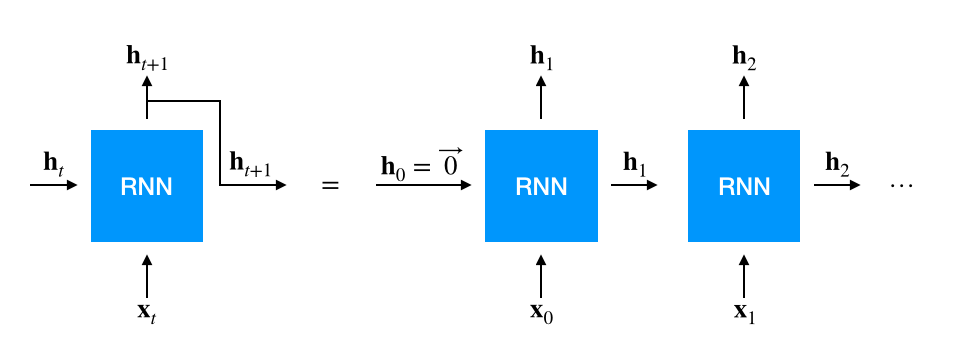Point forecasting methods take the hidden state and output a single point estimated value of the next time point. In time-series regression, the model is often trained by minimizing the mean squared error (MSE) of this output with respect to the ground truth value: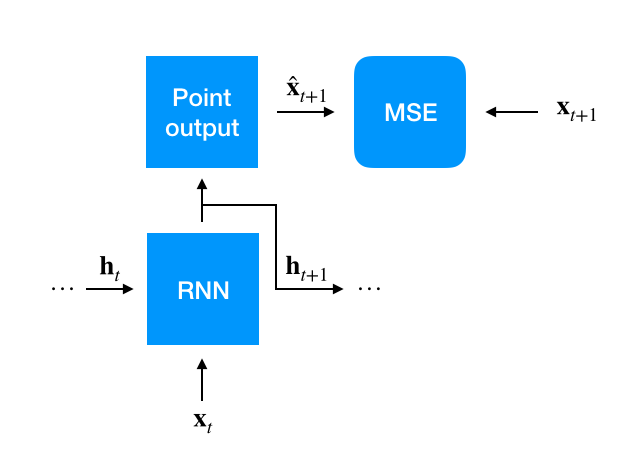When the model is used for prediction, we first run the RNN over the historical data available to warm it up. This state of the RNN is then continued into prediction times by feeding the outputs of the model at each timestep as input to the model for the next time step, in addition to the state: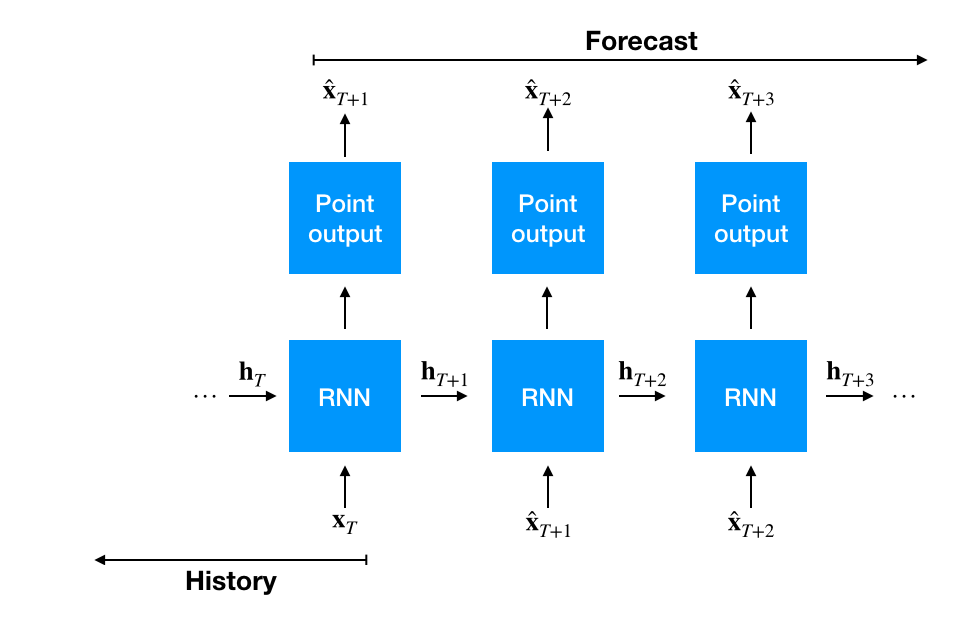### How can this method be generalized to probabilistic forecasts?

Instead of outputting a single value, like in point forecasting, parameters of a distribution must then be predicted: for example the mean and the variance of a Gaussian distribution. In the neural setting which we consider, this means a network is conditioned on the state coming from a RNN up to this time point, and this network then outputs distribution parameters. This model is then trained to minimize the negative log likelihood of the ground truth data with respect to the distribution parameters: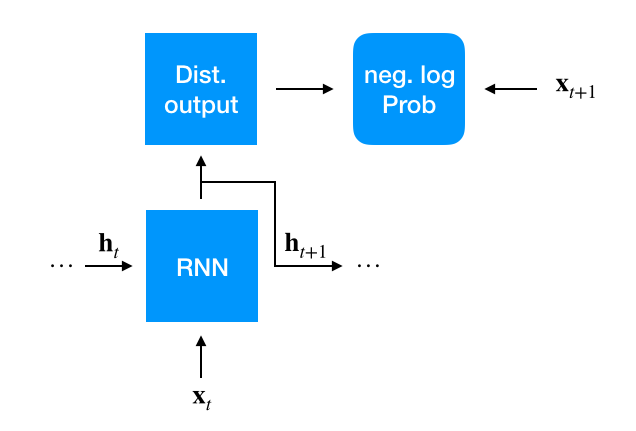In comparison to the deterministic point forecast at prediction time, we now have to sample a value from the output distribution for the next time step. This sample is then fed into the RNN to sample the next and so on. We can repeat this procedure as many times as we want, to get lots of sampled forecasts for the whole prediction interval. These samples can then be used to calculate statistical estimates, e.g. we can calculate any quantiles we are interested in: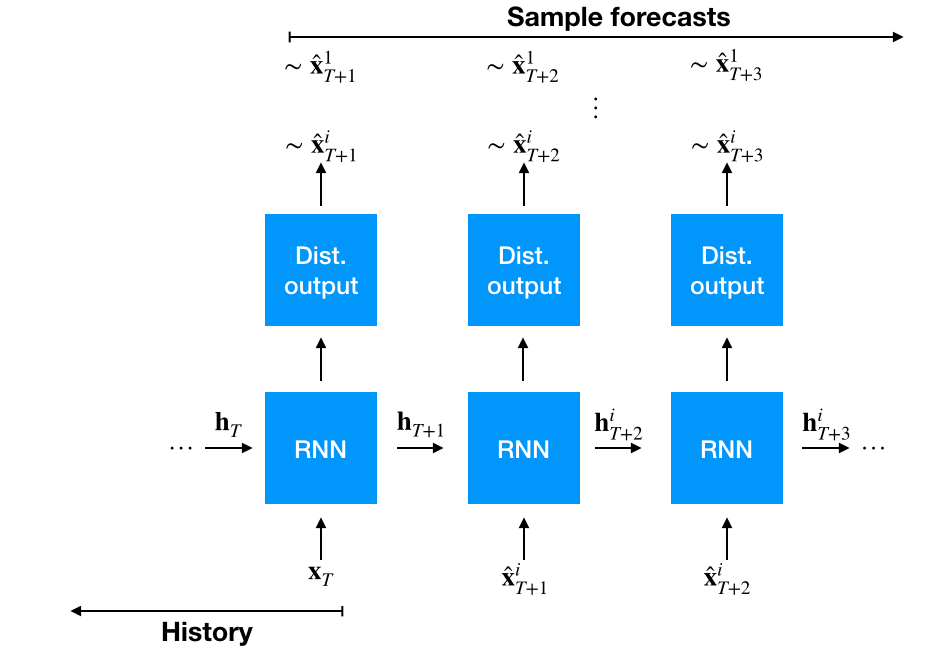### How does this process differ when we want to do multivariate forecasting?

Actually nothing much changes, at each time point as above the RNN now gets a multivariate vector, however, now the distribution has to account for the fact that we output a vector of values. We now need to learn the parameters of a multivariate distribution like a multivariate Gaussian.

Does this sufficiently capture multivariate dependencies? Well not really. For one, the number of parameters that make up the covariance matrix of a multivariate normal are quadratic in the size of the multivariate vector. And secondly, the calculation of the likelihood of a multivariate Gaussian distribution with respect to some ground truth data is cubic in the number of time series – hence, it doesn’t scale gracefully. One way to deal with this is to approximating the full multivariate Gaussian distribution by a low-rank approximation.

Furthermore, we are making a potentially strong assumption here: we select the output distribution for the kind of data we have to forecast (in the example we discussed we assumed a Gaussian distribution) before we even have seen the data. Is there a more data-driven way to determine this distribution? What would we need for such a method? For training, we would need the likelihood of the ground truth with respect to the distribution. And for the inference time, we would need to be able to sample from this distribution.

### Normalizing Flows

Normalizing flows are a model class that allow for efficient evaluation of the likelihood and efficient sampling. They are composition of invertible functions that transform samples from a complicated distribution to samples of a simple one like for example an isotropic Gaussian: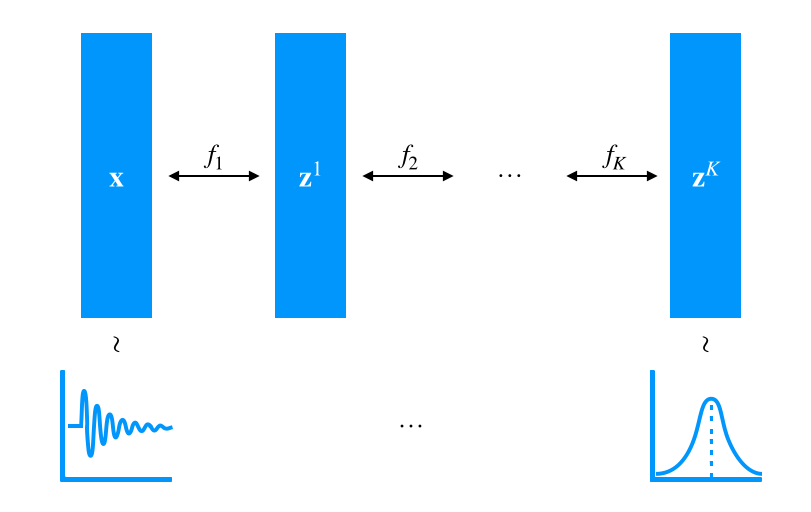Normalizing flows are in fact trainable neural network stacks which transform their inputs step by step (via the composition or stacking) in a computationally efficient manner. They not only let us calculate the likelihood of some data efficiently with respect to the simpler distribution (in fact that is how we train these flows) but due to their invertible nature we can generate a sample from the simple distribution and end up with a sample from the complex one!

Use of normalizing flows means that we do not need to assume that the underlying multivariate data follows a standard and simple distribution. Moreover, the computational efficiency of normalizing flow models such as Real NVP or MAF makes our approach readily scalable to a large number of data dimensions.

We combined Flow models with our RNN model, where instead of a multivariate distribution we plug in a normalizing flow module which is conditioned on the state of this RNN at each time point.  As demonstrated by the empirical results in our paper, combining normalizing flows with temporal conditioning coming from an RNN, or an attention mechanism, gives us a very powerful multivariate time series model. To the best of our knowledge, we achieve state-of-the-art results on a variety of real-world benchmarks, outperforming a number of recent competing approaches.